# How to use the COUNTA Function in Excel

In this article, we will learn How to use the COUNTA Function in Excel.

What do with empty cells in list to count

Usually in Excel, we use the COUNT function to count numbers in a list containing values. The COUNT function only counts the numbers in the list whereas sometimes we need to count the text, boolean, date or time values too in the same list along with numbers. But still wanted to ignore the blank cells in the list. Let's learn more about COUNTA function syntax and an example to illustrate the function usage.

COUNTA Function in Excel

COUNTA function is a built in Excel function to calculate the Count of cells having Text, numbers or logic_test.

Syntax:

 =COUNTA (value1, [value2], ...)

value1, [value2], … are values given as a list separated by comma or array reference (e.g. M10:M23).

 Note: Only Empty cells not considered by the COUNTA function

If we write a formula in Excel cell

 =COUNTA(1,2,"Computers","")

It will return 4 as the count of cells having values are 4.

Here "" creates an empty cell but is counted by COUNTA function.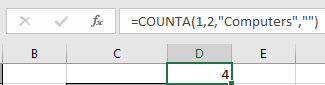Example :

All of these might be confusing to understand. Let's understand how to use the function using an example. Here we have some values in column A including the formula in A4 cell.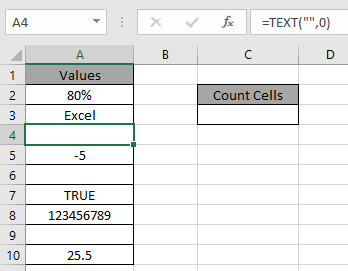Use the formula:

 =COUNTA(A2:A10)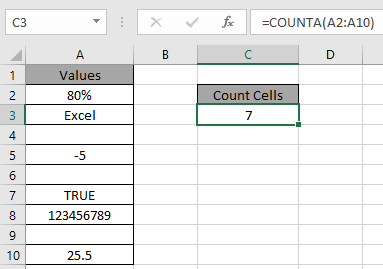7 is the count of numeric value cells. Fully Empty cells are A6 & A9.

Use of COUNTIFS function to understand the difference

All of these might be confusing to understand. So, let's test this formula via running it on the example shown below. Here we have the ID records and we need to find the COUNT of ID values where respective numbers lays between the given range.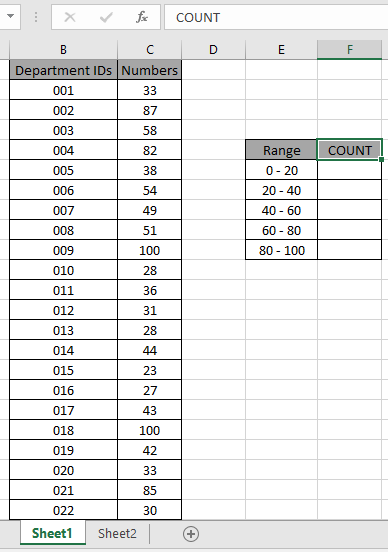Here to find the values in range. Some range references given using named range.

Numbers array given as Value named range for the array C3:C24.

Use the formula:

 = COUNTIFS ( Value , ">=0" , Value , "<20" )

Explanation:

• COUNTIFS function counts the cells that match the numbers in the Value array which are greater than equals to 0 (0 included).
• The COUNTIFS function counts the cells that match the numbers in the Value array which are less than 20 (20 excluded).
• The formula counts the cell which satisfies both conditions which means value which lay between 0 to 20 will be counted.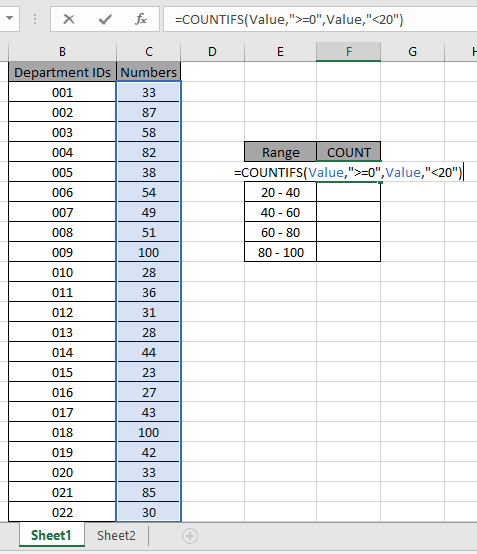The formula looks like as shown in the above snapshot. The Value array is given as a named range.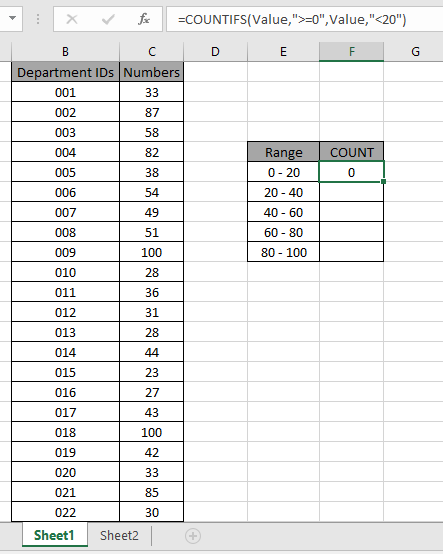As you can see the total values which occur between 0 - 20 comes out to be 0 i.e. there are no such values between 0 - 20.

Now copy the formula in other cells using the drag down option or using the shortcut key Ctrl + D as shown below. And changing the range parameter to 20 - 40.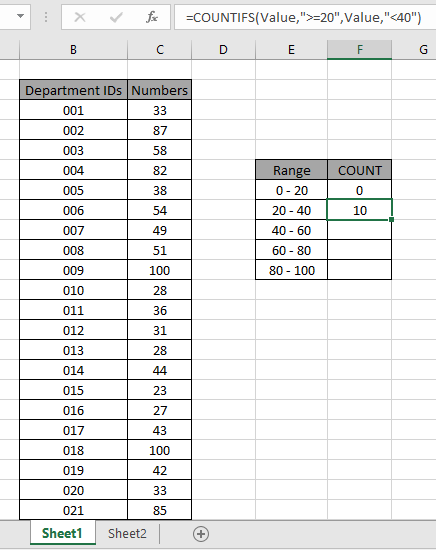As you can see values which lay between 20 - 40 are 10.

Now calculate all the other counts with given range values as explained above.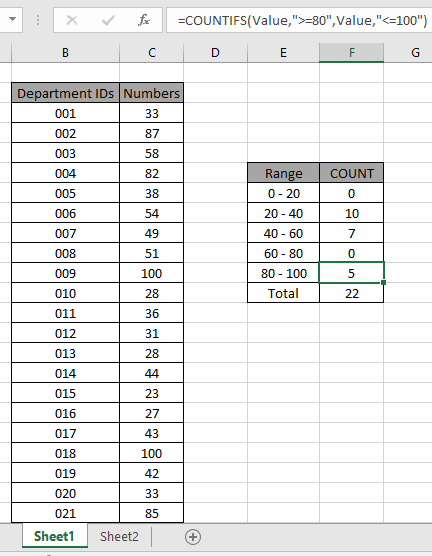We obtained all the count between given range as it also proves the formula works fine.

Use the COUNTA function to count text and logic_values in Excel.

Here are all the observational notes using the COUNTA function in Excel
Notes :

1. The COUNTA function returns the count as number value in the cell or can be used with other functions.
2. Use the array reference to select the list like values in array from M10 to M23 cell will be shown as M10:M23. Can add more values using comma as separator.

Hope this article about How to use the COUNTA function in Excel is explanatory. Find more articles on counting values and related Excel formulas here. If you liked our blogs, share it with your friends on Facebook. And also you can follow us on Twitter and Facebook. We would love to hear from you, do let us know how we can improve, complement or innovate our work and make it better for you. Write to us at info@exceltip.com.

Related Articles :

COUNTIFS with Dynamic Criteria Range : Count cells dependent on other cell values in Excel.

COUNTIFS Two Criteria Match : Count cells matching two different criteria on list in excel.

COUNTIFS With OR For Multiple Criteria : Count cells having multiple criteria match using the OR function.

The COUNTIFS Function in Excel : Count cells dependent on other cell values.

How to Use Countif in VBA in Microsoft Excel : Count cells using Visual Basic for Applications code.

How to use wildcards in excel : Count cells matching phrases using the wildcards in excel

How to use the SUMPRODUCT function in Excel: Returns the SUM after multiplication of values in multiple arrays in excel.

How to use wildcards in excel : Count cells matching phrases using the wildcards in excel

Popular Articles :

How to use the IF Function in Excel : The IF statement in Excel checks the condition and returns a specific value if the condition is TRUE or returns another specific value if FALSE.

How to use the VLOOKUP Function in Excel : This is one of the most used and popular functions of excel that is used to lookup value from different ranges and sheets.

How to use the SUMIF Function in Excel : This is another dashboard essential function. This helps you sum up values on specific conditions.

How to use the COUNTIF Function in Excel : Count values with conditions using this amazing function. You don't need to filter your data to count specific values. Countif function is essential to prepare your dashboard.

Terms and Conditions of use

The applications/code on this site are distributed as is and without warranties or liability. In no event shall the owner of the copyrights, or the authors of the applications/code be liable for any loss of profit, any problems or any damage resulting from the use or evaluation of the applications/code.